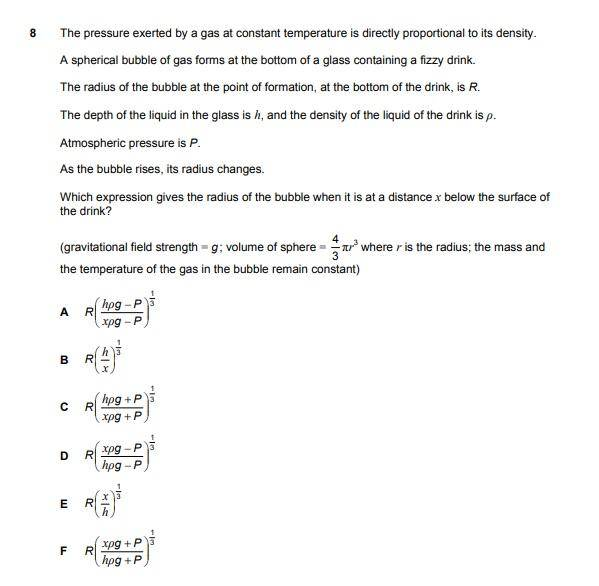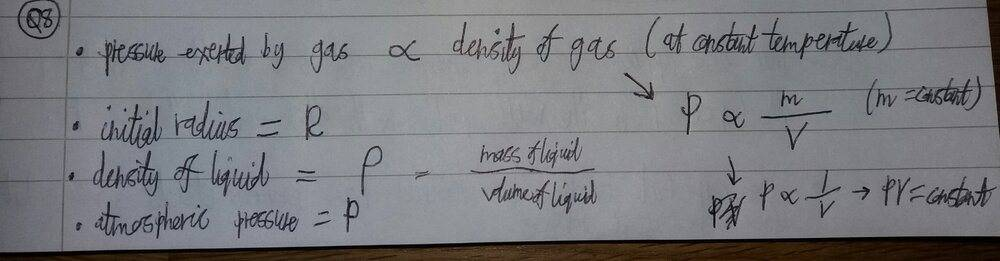# Pressure of a gas bubble

TomK
Homework Statement:
ENGAA 2019 (Q8, Section 2)
Relevant Equations:
Pressure Equations
Ideal Gas LawsI'm having trouble understanding the solution shown on this link (http://www.engineeringadmissionsassessment.com/2019-solutions.html) - scroll down to Section 2, Question 8.

From what I've gathered, [final pressure = initial pressure x 'R^3/r^3'], as PV must be constant. Therefore, I understand why they equate 'R^3/r^3' to 'final pressure/initial pressure'.

However, why doesn't the pressure exerted by the gas (within the bubble) affect the values of the initial and final pressure, since the bubble's volume is changing as it rises? Doesn't the pressure exerted by the gas (outward) oppose the pressure exerted on the gas (inward) by the liquid and atmospheric pressure?

In the solution, it looks like their initial/final pressure values only account for the 'pressure due to being submerged' and 'atmospheric pressure'. This is what I'm getting confused by.

Homework Helper
Doesn't the pressure exerted by the gas (outward) oppose the pressure exerted on the gas (inward) by the liquid and atmospheric pressure?
Newton's third law.

The outward pressure of the gas in the bubble on the fluid outside is exactly equal to the inward pressure of the fluid outside on the gas inside.

The two forces are not "opposed". They are part and parcel of the same force pair.

TomK
Newton's third law.

The outward pressure of the gas in the bubble on the fluid outside is exactly equal to the inward pressure of the fluid outside on the gas inside.

The two forces are not "opposed". They are part and parcel of the same force pair.

So, as the bubble rises from the base to the given height, the bubble changes volume, in order for the pressure outside = pressure inside?

Homework Helper
So, as the bubble rises from the base to the given height, the bubble changes volume, in order for the pressure outside = pressure inside?
Yes.

If pressure inside had remained the same while pressure outside declined, the fluid at the boundary would be under an unbalanced net force. The bubble would expand, forcing that fluid outward.

Which is exactly what happens. The fluid is forced outward, the bubble expands, its volume increases and its pressure declines so that a new equilibrium is attained.

TomK
Yes.

If pressure inside had remained the same while pressure outside declined, the fluid at the boundary would be under an unbalanced net force. The bubble would expand, forcing that fluid outward.

Which is exactly what happens. The fluid is forced outward, the bubble expands, its volume increases and its pressure declines so that a new equilibrium is attained.

Thank you for helping.